search
Search
Unlock 100+ guides
search toc
close
account_circle
Profile
exit_to_app
Sign out
search
keyboard_voice
close
Searching Tips
Search for a recipe:
"Creating a table in MySQL"
Search for an API documentation: "@append"
Search for code: "!dataframe"
Apply a tag filter: "#python"
Useful Shortcuts
/ to open search panel
Esc to close search panel
to navigate between search results
d to clear all current filters
Enter to expand content previewDoc SearchCode Search BetaSORRY NOTHING FOUND!
mic
Start speaking...Voice search is only supported in Safari and Chrome.
Shrink
Navigate to

# Guide on System of Linear Equations in Linear Algebra

schedule Aug 12, 2023
Last updated
local_offer
Linear Algebra
Tags
mode_heat
Master the mathematics behind data science with 100+ top-tier guides
Start your free 7-days trial now!
Definition.

# Linear equation

An equation is said to be a linear equation with $n$ variables $x_1$, $x_2$, $\cdots$, $x_n$ if and only if the equation has the following form:

$$a_1x_1+a_2x_2+\cdots+a_nx_n=b$$

Where:

• $a_1$, $a_2$, $\cdots$, $a_n$ and $b$ are some constants.

• at least one of $a_1$, $a_2$, $\cdots$, $a_n$ is non-zero.

The variables $x_1$, $x_2$, $\cdots$, $x_n$ are also known as unknowns, and $b$ is sometimes referred to as the constant term.

Example.

## Linear equations

The following is an example of a linear equation with two variables:

$$3x_1+x_2=5$$

Note the following:

• when working with two variables, we often denote the variables as $x$ and $y$.

• when working with three variables, we often denote the variables as $x$, $y$ and $z$.

The following is an example of a linear equation with three variables:

$$x+4y+5z=9$$
Example.

## Non-linear equations

The following are some examples of non-linear equations:

\begin{align*} x+4xy&=3\\ \sin(x)+xy&=4\\ x^2+y&=5\\ x+\sqrt{y}&=6\\ \end{align*}

These are non-linear because the variables $x_1$, $x_2$, $\cdots$, $x_n$ in a linear equation must be of the following form by definition:

$$a_1x_1+a_2x_2+\cdots+a_nx_n=b$$

# Graphical representation of a linear equation

Recall that an equation of a line in two-dimensional space is given by:

$$ax+by=c$$

Where $a$, $b$ and $c$ are constants. This is a linear equation with two variables $x$ and $y$. Given the constant terms, we can visualize the linear equation. For instance, the $2x+3y=4$ can be visualized by the following plot: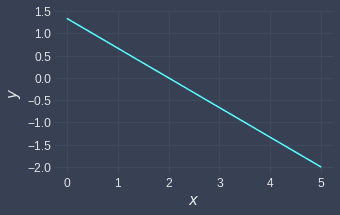Similarly, a linear equation with three variables $x$, $y$ and $z$ can also be graphed. For example, the graph of the linear equation $2x+3y+4z=5$ is:As we can see, the linear equation with $3$ variables is a flat plane when visualized.

The graphs of the linear equations motivate why linear equations are described as "linear" - their graphs are straight and non-curvy. In contrast, a non-linear equation such as $2x^2+3y=4$ will take on a curvy shape: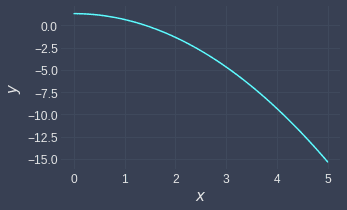Definition.

# Homogeneous linear equation

A homogeneous linear equation with $n$ variables $x_1$, $x_2$, $\cdots$, $x_n$ has the following form:

$$a_1x_1+a_2x_2+\cdots+a_nx_n=0$$

A homogeneous linear system is a collection of homogeneous linear equations - for instance:

$$\begin{cases} 2x+3y&=0\\ 4x+y&=0\\ \end{cases}$$

We will later cover some applications of homogenous linear equations.

Definition.

# System of linear equations

A finite set of linear equations with $n$ variables is called a system of linear equations or simultaneous equations:

\begin{align*} a_{1,1}x_1+\;a_{1,2}x_2+\cdots+\;a_{1,n}x_n&=b_1\\ a_{2,1}x_1+\;a_{2,2}x_2+\cdots+\;a_{2,n}x_n&=b_2\\ \vdots\;\;\;\;\;\;\;\;\;\;\;\;\;\;\;\;\vdots\;\;\;\;\;\;\;\;\;\;\;\;\;\;\;\;\vdots\\ a_{m,1}x_1+a_{m,2}x_2+\cdots+a_{m,n}x_n&=b_m\\ \end{align*}

A solution of a system of linear equations is the values that the variables $x_1$, $x_2$, $\cdots$, $x_n$ take on to make all the above equalities hold.

Example.

## Computing a solution of a system of linear equations

Compute the solution of the following simultaneous equations:

$$\begin{cases} 3x+y=5\\ x+y=1\\ \end{cases}$$

Solution. Subtracting the bottom equation from the top equation yields:

\begin{align*} 2x&=4\\ x&=2 \end{align*}

Substituting $x=2$ into the bottom equation:

\begin{align*} 2+y&=1\\ y&=-1 \end{align*}

Therefore, the solution of the simultaneous equations is the pair $x=2$ and $y=-1$.

Definition.

# Consistent and inconsistent system of linear equations

A consistent system of linear equations has either one solution or infinitely many solutions. An inconsistent system of linear equations has no solution.

Example.

## Inconsistent system with no solution

Consider the following system of linear equations:

$$\begin{cases} y=x+2\\ y=x+4\\ \end{cases}$$

Show that this system is inconsistent.

Solution. Subtracting the second equation from the first equation yields:

$$0=-2$$

We get a contradiction here, which means that there exists no $(x,y)$ pair that satisfy both our linear equations. Because there exists no solution, our linear system of equations is inconsistent.

Let's graph the two equations: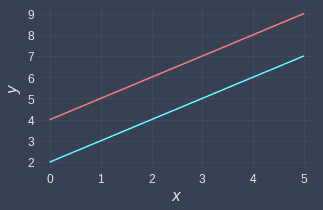As we can see, the lines are parallel to each other and thus never intersect.

Example.

## Consistent system with a single solution

Consider the following system of linear equations:

$$\begin{cases} y=2x+2\\ y=x+4\\ \end{cases}$$

Show that this system is consistent.

Solution. Subtracting the second equation from the first equation:

\begin{align*} 0&=x-2\\ x&=2 \end{align*}

Substituting the value of $x$ into the top equation:

\begin{align*} y&=2(2)+2\\ &=6 \end{align*}

Since a solution exists, this system of linear equations is consistent.

Let's graph the two equations: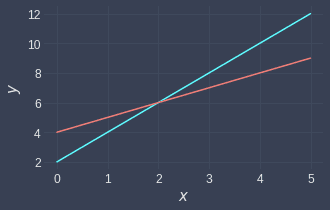We can see that the two lines intersect at a single point $(2,6)$, just as we calculated! We sometimes refer to the intersection point as a unique solution.

Example.

## Consistent system with infinitely many solutions

Consider the following system of linear equations:

$$\begin{cases} x+y=2\\ 2x+2y=4\\ \end{cases}$$

Show that this system is consistent.

Solution. Multiplying the top equation by $2$ and then subtracting the bottom equation from the top equation:

$$0=0$$

This tells us that the top and bottom equations are equivalent! Because there are infinitely many intersection points between the two identical equations, there are infinitely many solutions. This means that the system is consistent.

Let's plot the two equations: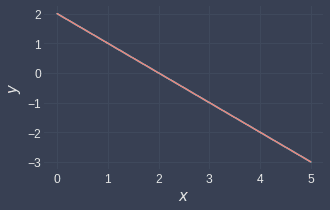We only see one line here because the two equations overlap with one another. Every point on this line is a solution.

Theorem.

# Matrix form of system of linear equations

All systems of linear equations can be expressed in the following matrix form:

$$\boldsymbol{Ax}=\boldsymbol{b}$$

Where:

• $\boldsymbol{A}\in\mathbb{R}^{m\times{n}}$ is the matrix holding the coefficients of the variables.

• $\boldsymbol{x}\in\mathbb{R}^n$ is a vector holding the variables $x_1$, $x_2$, $\cdots$, $x_n$.

• $\boldsymbol{b}\in\mathbb{R}^m$ is a vector holding the constant terms $b_1$, $b_2$, $\cdots$, $b_m$.

Expressing a system of linear equations in matrix form allows us to use a technique called Gaussian Elimination to find the solutions.

Sketch proof. Consider the following system of linear equations:

$$\begin{cases} x+2y=5\\ 4+3y=6\\ \end{cases}$$

This can be written in the following matrix form:

$$\begin{pmatrix} 1&2\\ 4&3 \end{pmatrix} \begin{pmatrix} x\\y \end{pmatrix}= \begin{pmatrix} 5\\6 \end{pmatrix}$$

Performing the matrix-vector multiplication on the left:

$$\begin{pmatrix} x+2y\\ 4x+3y \end{pmatrix} = \begin{pmatrix} 5\\6 \end{pmatrix}$$

Equating the components gives us the original system of linear equations.

Here's another example - consider the following simultaneous equation:

$$\begin{cases} x_1+2x_2+4x_3=5\\ x_1+3x_2+2x_3=6\\ 2x_1+x_2+5x_3=0\\ \end{cases}$$

The corresponding matrix form is:

$$\begin{pmatrix} 1&2&4\\ 1&3&2\\ 2&1&5 \end{pmatrix} \begin{pmatrix} x_1\\x_2\\x_3 \end{pmatrix}= \begin{pmatrix} 5\\6\\0 \end{pmatrix}$$

We often denote:

• the matrix containing the coefficients as $\boldsymbol{A}$.

• the vector containing the variables as $\boldsymbol{x}$.

• the vector on the right as $\boldsymbol{b}$.

Therefore, the short-hand notation of the matrix form of a system of linear equations is:

$$\boldsymbol{Ax}=\boldsymbol{b}$$

This completes the sketch proof.

Example.

## Expressing a system of linear equations in matrix form

Express the following system of linear equations in matrix form:

$$\begin{cases} x+2y+5z&=5\\ 4x+3y+3z&=6\\ 2x+y+4z&=0\\ \end{cases}$$

Solution. The system of linear equation in matrix form is:

$$\begin{pmatrix} 1&2&5\\ 4&3&3\\ 2&1&4 \end{pmatrix} \begin{pmatrix} x\\y\\z \end{pmatrix}= \begin{pmatrix} 5\\6\\0 \end{pmatrix}$$

The following theorem is useful for future proofs - feel free to skip it for now and come back later when referenced.

Theorem.

# Expressing a system of vector equations as a matrix product

Consider the following vector equations:

$$\begin{equation}\label{eq:buhgshTVAp7jnzGG7E2} \begin{gathered} \boldsymbol{v}_1 =b_{11}\boldsymbol{a}_1+b_{12}\boldsymbol{a}_2+\cdots+b_{1n}\boldsymbol{a}_n\\ \boldsymbol{v}_2 =b_{21}\boldsymbol{a}_1+b_{22}\boldsymbol{a}_2+\cdots+b_{2n}\boldsymbol{a}_n\\ \vdots\\ \boldsymbol{v}_m =b_{m1}\boldsymbol{a}_1+b_{m2}\boldsymbol{a}_2+\cdots+b_{mn}\boldsymbol{a}_n\\ \end{gathered} \end{equation}$$

Where:

• $\boldsymbol{v}_1, \boldsymbol{v}_2,\cdots, \boldsymbol{v}_m\in\mathbb{R}^k$.

• $\boldsymbol{a}_1,\boldsymbol{a}_2,\cdots,\boldsymbol{a}_n\in \mathbb{R}^k$.

• $b_{ij}\in\mathbb{R}$ for $1\le{i}\le{m}$ and $1\le{j}\le{n}$.

The equations in \eqref{eq:buhgshTVAp7jnzGG7E2} hold if and only if the following holds:

$$\begin{pmatrix} \vert&\vert&\cdots&\vert\\ \boldsymbol{v}_1&\boldsymbol{v}_2&\cdots&\boldsymbol{v}_m\\ \vert&\vert&\cdots&\vert\\ \end{pmatrix}= \begin{pmatrix} \vert&\vert&\cdots&\vert\\ \boldsymbol{a}_1&\boldsymbol{a}_2&\cdots&\boldsymbol{a}_n\\ \vert&\vert&\cdots&\vert\\ \end{pmatrix} \boldsymbol{B}^T$$

Where $\boldsymbol{B}$ is an $m\times{n}$ matrix:

$$\boldsymbol{B}= \begin{pmatrix}b_{11}&b_{12}&\cdots&b_{1n}\\ b_{21}&b_{22}&\cdots&b_{2n}\\ \vdots&\vdots&\smash\ddots&\vdots\\ b_{m1}&b_{m2}&\cdots&b_{mn}\end{pmatrix}$$

Proof. Suppose we have the following vector equations:

$$\begin{equation}\label{eq:hHq8hy4pXBtw3QbSv62} \begin{gathered} \boldsymbol{v}_1 =b_{11}\boldsymbol{a}_1+b_{12}\boldsymbol{a}_2+\cdots+b_{1n}\boldsymbol{a}_n\\ \boldsymbol{v}_2 =b_{21}\boldsymbol{a}_1+b_{22}\boldsymbol{a}_2+\cdots+b_{2n}\boldsymbol{a}_n\\ \vdots\\ \boldsymbol{v}_m =b_{m1}\boldsymbol{a}_1+b_{m2}\boldsymbol{a}_2+\cdots+b_{mn}\boldsymbol{a}_n\\ \end{gathered} \end{equation}$$

Define an $k\times{n}$ matrix $\boldsymbol{A}$ and an $m\times{n}$ matrix $\boldsymbol{B}$ like so:

$$\boldsymbol{A}=\begin{pmatrix} \vert&\vert&\cdots&\vert\\ \boldsymbol{a}_1&\boldsymbol{a}_2&\cdots&\boldsymbol{a}_n\\ \vert&\vert&\cdots&\vert\\ \end{pmatrix},\;\;\;\;\; \boldsymbol{B}= \begin{pmatrix}b_{11}&b_{12}&\cdots&b_{1n}\\ b_{21}&b_{22}&\cdots&b_{2n}\\ \vdots&\vdots&\smash\ddots&\vdots\\ b_{m1}&b_{m2}&\cdots&b_{mn}\end{pmatrix}= \begin{pmatrix} -&\boldsymbol{b}_1&-\\ -&\boldsymbol{b}_2&-\\ \vdots&\vdots&\vdots\\ -&\boldsymbol{b}_m&-\\ \end{pmatrix}$$

The transpose $\boldsymbol{B}^T$ can be expressed as a collection of column vectors:

$$\boldsymbol{B}=\begin{pmatrix} \vert&\vert&\cdots&\vert\\ \boldsymbol{b}_1&\boldsymbol{b}_2&\cdots&\boldsymbol{b}_m\\ \vert&\vert&\cdots&\vert\\ \end{pmatrix}$$

$$\begin{equation}\label{eq:AScL9hXe2XbDLR4P2GH} \boldsymbol{A}\boldsymbol{B}^T= \boldsymbol{A} \begin{pmatrix} \vert&\vert&\cdots&\vert\\ \boldsymbol{b}_1&\boldsymbol{b}_2&\cdots&\boldsymbol{b}_m\\ \vert&\vert&\cdots&\vert\\ \end{pmatrix} = \begin{pmatrix} \vert&\vert&\cdots&\vert\\ \boldsymbol{Ab}_1&\boldsymbol{Ab}_2&\cdots&\boldsymbol{Ab}_m\\ \vert&\vert&\cdots&\vert\\ \end{pmatrix} \end{equation}$$

Here, the column vectors of $\boldsymbol{B}^T$ are:

$$\boldsymbol{b}_1= \begin{pmatrix} b_{11}\\b_{12}\\\vdots\\b_{1n} \end{pmatrix},\;\;\;\;\; \boldsymbol{b}_2= \begin{pmatrix} b_{21}\\b_{22}\\\vdots\\b_{2n} \end{pmatrix},\;\;\;\;\; \cdots\;\;\;\;\;,\;\;\;\;\; \boldsymbol{b}_{m}=\begin{pmatrix} b_{m1}\\b_{m2}\\\vdots\\b_{mn} \end{pmatrix}$$

By theoremlink, every column in \eqref{eq:AScL9hXe2XbDLR4P2GH} can be expressed as:

\begin{align*} \boldsymbol{Ab}_1&= \begin{pmatrix} \vert&\vert&\cdots&\vert\\ \boldsymbol{a}_1&\boldsymbol{a}_2&\cdots&\boldsymbol{a}_n\\ \vert&\vert&\cdots&\vert\\ \end{pmatrix}\begin{pmatrix} b_{11}\\b_{12}\\\vdots\\b_{1n} \end{pmatrix} =b_{11}\boldsymbol{a}_1+ b_{12}\boldsymbol{a}_2+\cdots+b_{1n}\boldsymbol{a}_n\\ \boldsymbol{Ab}_2&= \begin{pmatrix}\vert&\vert&\cdots&\vert\\ \boldsymbol{a}_1&\boldsymbol{a}_2&\cdots&\boldsymbol{a}_n\\ \vert&\vert&\cdots&\vert\\\end{pmatrix}\begin{pmatrix} b_{21}\\b_{22}\\\vdots\\b_{2n} \end{pmatrix} =b_{21}\boldsymbol{a}_1+ b_{22}\boldsymbol{a}_2+\cdots+b_{2n}\boldsymbol{a}_n\\\\ &\;\;\;\;\;\;\;\;\;\;\;\;\;\;\;\;\;\;\;\;\;\;\;\;\;\;\;\;\;\;\;\;\;\;\;\;\;\;\;\;\;\;\;\;\vdots\\\\ \boldsymbol{Ab}_m&= \begin{pmatrix}\vert&\vert&\cdots&\vert\\ \boldsymbol{a}_1&\boldsymbol{a}_2&\cdots&\boldsymbol{a}_n\\ \vert&\vert&\cdots&\vert\\\end{pmatrix}\begin{pmatrix} b_{m1}\\b_{m2}\\\vdots\\b_{mn} \end{pmatrix} =b_{m1}\boldsymbol{a}_1+ b_{m2}\boldsymbol{a}_2+\cdots+b_{mn}\boldsymbol{a}_n \end{align*}

Comparing this with \eqref{eq:hHq8hy4pXBtw3QbSv62} gives:

$$\begin{equation}\label{eq:B6zF8q6lmCMEYXUI64X} \begin{gathered} \boldsymbol{v}_1 =\boldsymbol{Ab}_1\\ \boldsymbol{v}_2 =\boldsymbol{Ab}_2\\ \vdots\\ \boldsymbol{v}_m =\boldsymbol{Ab}_m\\ \end{gathered} \end{equation}$$

Therefore, \eqref{eq:AScL9hXe2XbDLR4P2GH} can be written as:

$$\boldsymbol{A}\boldsymbol{B}^T= \begin{pmatrix} \vert&\vert&\cdots&\vert\\ \boldsymbol{a}_1&\boldsymbol{a}_2&\cdots&\boldsymbol{a}_n\\ \vert&\vert&\cdots&\vert\\ \end{pmatrix}\boldsymbol{B}^T =\begin{pmatrix} \vert&\vert&\cdots&\vert\\ \boldsymbol{v}_1&\boldsymbol{v}_2&\cdots&\boldsymbol{v}_m\\ \vert&\vert&\cdots&\vert\\ \end{pmatrix}$$

This completes the proof.

thumb_up
thumb_down
Comment
Citation
Ask a question or leave a feedback...
thumb_up
0
thumb_down
0
chat_bubble_outline
0
settings
Enjoy our search
Hit / to insta-search docs and recipes!